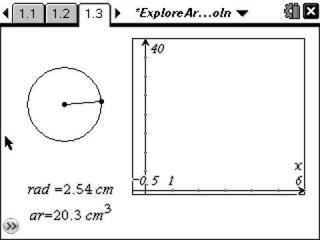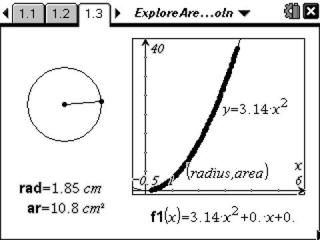# Activities

••• ##### Subject Area

• Math: Geometry: Circles

• ##### Author9-12

15 Minutes

• ##### Device
•TI-Nspire™ CX/CX II
•TI-Nspire™ CX CAS/CX II CAS
• TI-Nspire™ Navigator™
• TI-Nspire™
• TI-Nspire™ CAS
• ##### Software

TI-Nspire™
TI-Nspire™ CAS

2.1

• ##### Report an Issue

Exploring the Area of a Circle

#### Activity Overview

Students explore the relationship between the area and radius of a circle.

#### Key Steps

•Students investigate the area of a circle by creating a scatterplot for the radius and area data of a circle.

•Students graph the values captured and stored in the spreadsheet. They then graph y = x² and transform it to fit their data.

Students then find the equation of the parabola. They will see it as y = 3.14(x)2. Students then find the quadratic regression to verify their equation.

At the end of this activity, students will be able to verify the circumference and area formulas for a circle using technology.